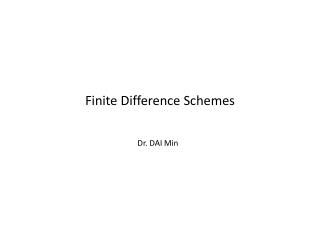Download PresentationFinite Difference Schemes

# Finite Difference Schemes

Download Presentation## Finite Difference Schemes

- - - - - - - - - - - - - - - - - - - - - - - - - - - E N D - - - - - - - - - - - - - - - - - - - - - - - - - - -
##### Presentation Transcript

1. Finite Difference Schemes Dr. DAI Min

2. Type of finite difference scheme Explicit scheme Advantage There is no need to solve a system of algebraic equations Easy for programming Disadvantage: conditionally convergent Implicit scheme Fully implicit scheme: first order accuracy Crank-Nicolson scheme: second order accuracy

3. Explicit scheme European put option: Lattice:

4. Explicit scheme (continued)

5. Explicit scheme (continued)

6. Explicit scheme (continued)

7. Explicit scheme (continued) Monotone scheme

8. Explicit scheme for a transformed equation Transformed Black-Scholes equation:

9. Explicit scheme for a transformed equation

10. Explicit scheme for a transformed equation (continued)

11. Explicit scheme for a transformed equation (continued)

12. Equivalence of explicit scheme and BTM

13. Equivalence of explicit scheme and BTM (continued)

14. Why use implicit scheme? • Explicit scheme is conditionally convergent

15. Fully implicit scheme

16. Fully implicit scheme (continued)

17. Matrix form of an explicit scheme

18. Monotonicity of the fully implicit scheme

19. Second-order scheme: Crank-Nicolson scheme

20. Crank-Nicolson scheme in matrix form

21. Convergence of Crank-Nicolson scheme • The C-N scheme is not monotone unless  t/h2 is small enough. • Monotonicity is sufficient but not necessary • The unconditional convergence of the C-N scheme (for linear equation) can be proved using another criterion (see Thomas (1995)). • Due to lack of monotonicity, the C-N scheme is not as stable/robust as the fully implicit scheme when dealing with tough problems.

22. Iterative methods for solving a linear system

23. Linearization for nonlinear problems

24. Newton iteration

25. Handling non-smooth terminal conditions • C-N scheme has a better accuracy but is unstable when the terminal condition is non-smooth. • To cure the problem • Rannacher smoothing • Smoothing the terminal value condition

26. Upwind (upstream) treatment

27. An example for upwind scheme in finance

28. Artificial boundary conditions • Solution domain is often unbounded, but implicit schemes should be restricted to a bounded domain • Truncated domain • Change of variables • Artificial boundary conditions should be given based on • Properties of solution, and/or • PDE with upwind scheme

29. Examples • European call options • CIR model for zero coupon bond

30. CIR models (continued) • Method 1: confined to [0,M] • Method 2: a transformation

31. Test of convergence order

32. Test of convergence order (alternative method)

33. An example: given benchmark values

34. An example: no benchmark values